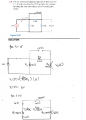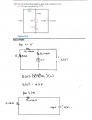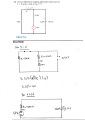# Voltage Across A RC Circuit (for t > 0)

#### libnitz

Joined Jan 29, 2016
17Could someone please explain whats going on for t > 0. For t = 0-, I understand that the capacitor turns into an open circuit and Voc is straightforward to calculate. However, for t > 0, it appears the 12 V voltage supply was removed from the figure in order to find the voltage across the capacitor. Why was the 12 V voltage supply removed from figure.

A similar problem;In this situation, I'm confused as to why the R3 = 4k ohms and the 6 V power supply were removed in order to find the Voltage across the capacitor for t > 0 but the R1 = 3 k ohms is included.

Now lastly this problem,It looks slightly different than the previous 2 problems because in this situation, the 12 V power supply is included in the circuit diagram to calculate Vc but yet again the R1 = 3 k ohms is included.

All three situations have caused a difficult conundrum I am battling with as to why for t > 0 some parts of the circuits are cut off.

Any help is greatly appreciated.
Thanks.

#### Jony130

Joined Feb 17, 2009
5,457
At t = 0 the switch is open and this is why 12V source or 4V source together with 4k resistor are remove form the circuit and in your last problem we remove 3k resistor because switch is open at t =0.
Also notice that the capacitor is charge to the voltage present across it at t = 0-. And at time t = 0 the capacitor him self is act just like a voltage source.

•libnitz